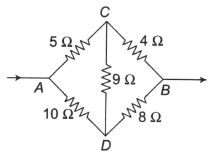Five resistors are connected as shown in the diagram. The equivalent resistance between A and B is

# Five resistors are connected as shown in the diagram. The equivalent resistance between A and B is1. A

6 ohm

2. B

9 ohm

3. C

12 ohm

4. D

15 ohm

Register to Get Free Mock Test and Study Material

+91

Verify OTP Code (required)

### Solution:

Since the given bridge is balanced, hence there will be no current through $9\text{\hspace{0.17em}\hspace{0.17em}}\Omega$ resistance. This resistance has no effect and must be ignored in the calculations.

${\mathrm{R}}_{\mathrm{AB}}=\text{\hspace{0.17em}}\frac{9\text{\hspace{0.17em}}×\text{\hspace{0.17em}}18}{27}\text{\hspace{0.17em}\hspace{0.17em}}=\text{\hspace{0.17em}}6\text{\hspace{0.17em}\hspace{0.17em}}\mathrm{\Omega }$Register to Get Free Mock Test and Study Material

+91

Verify OTP Code (required)